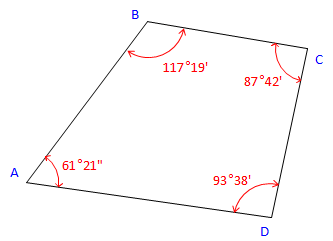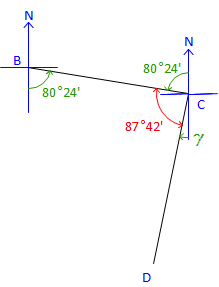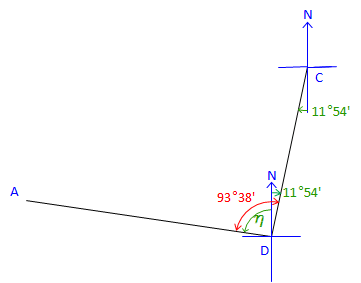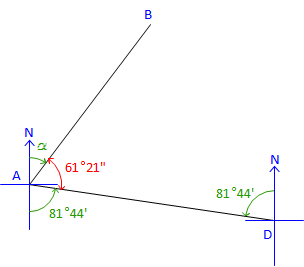## 2. Bearings From Interior Angles

Given the following traverse and horizontal angles:Figure C-23 Bearing Traverse

Using a bearing of N 36°55' E for line AB, determine the bearings of the remaining lines clockwise around the traverse.

Many survey texts use a tabular approach to compute traverse line directions from angles. For the beginner this can be confusing and lead to erroneous directions. It helps to instead draw sketches in order to visualize the line relationships.

 At point B:Figure C-24 Label the bearing angle, 36°55', from B to A. Subtract it from 117°19'. β = 117°19' - 36°55' = 80°24'Brg BC = S 80°24' E At point C:Figure C-25 Label the bearing angle, 80°24', from C to B. Subtract it along with 87°42' from 180°00' to get bearing angle CD. γ = 180°00' - (80°24' + 87°42') = 11°54' Brg CD = S 11°54' W At point D:Figure C-26 Label the bearing angle, 11°54', from D to C. Subtract it from 93°38' to obtain next bearing angle. &eta= 93°38' - 11°54' = 81°44'Brg DA = N 81°44' W The directions for all four traverse lines have been computed. Angles at B, C, and D have been used, but that at A has not. For a math check, use the Bearing of DA and the angle at A to compute the bearing we started with.Figure C-27 Label the bearing angle, 81°44', from A to D. Subtract it along with 61°21' from 180°00' to get next bearing angle. α = 180°00' - (61°21' + 81°44') = 36°55 Brg AB = N 36°55' E  check

If our computed and initial bearings for AB don’t match it means one of two things:

• there is a math error in our computations, or,
• the interior angles weren’t balanced.

For this traverse the angles sum to 360°00' so there is no angular misclosure. If our math check had failed it would have been due to a math error in our computations.

Had the angles not been balanced and if there were no math errors, the math check would be off by the angular misclosure.

Summary:

 Line Bearing AB N 36°55' E BC S 80°24' E CD S 11°54' W DA N 81°44' W